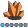hamed_jabarian
Calcite | Level 5

## How can i produce syntax in IML9.2 equivalent to " NORM.INV " in excel?

I am striving to calculate Value at risk in financial market, in order to produce 10% VaR in excel it is easy to use Norm.inv(probability,mean,std). what is the same code in IML?

Furthermore in excel we have " small(array,3) " to calculate 3rd smallest value. how is it done in IML?

1 ACCEPTED SOLUTION

Accepted Solutions

## Re: How can i produce syntax in IML9.2 equivalent to " NORM.INV " in excel?

The inverse CDF function is called the QUANTILE function in SAS. To compute the inverse CDF of the normal distribution, use the following:

proc iml;

prob=0.5; mean=0; std=1;

q = quantile("Normal", prob, mean, std);

For computing the kth smallest, I'd use the RANK function., which returns the order of each element in a sorted list.  You can use the LOC function to find the index that contains the kth smallest, and then extract the value, as follows:

x = 100:91;

r = rank(x);

idx = loc(r=3);   /* location of the 3rd smallest */

small3 = x[idx];  /* value of the 3rd smallest */

7 REPLIES 7

## Re: How can i produce syntax in IML9.2 equivalent to " NORM.INV " in excel?

The inverse CDF function is called the QUANTILE function in SAS. To compute the inverse CDF of the normal distribution, use the following:

proc iml;

prob=0.5; mean=0; std=1;

q = quantile("Normal", prob, mean, std);

For computing the kth smallest, I'd use the RANK function., which returns the order of each element in a sorted list.  You can use the LOC function to find the index that contains the kth smallest, and then extract the value, as follows:

x = 100:91;

r = rank(x);

idx = loc(r=3);   /* location of the 3rd smallest */

small3 = x[idx];  /* value of the 3rd smallest */Ksharp
Super User

## Re: How can i produce syntax in IML9.2 equivalent to " NORM.INV " in excel?

Rick,

What if there are some ties ? How to get rid of them ?

```proc iml;
m = { 1 2 0,
2 4 0,
10 11 12,
2 2 2};
r=rank(m);
x=loc(r=3);
smallest3=m;
print smallest3;
quit;

```

Xia Keshan

## Re: How can i produce syntax in IML9.2 equivalent to " NORM.INV " in excel?

Why would I want to get rid of them? The algorithm returns the observations with the k_th smallest values.  If you just want one, you can use

smallest3=m;Ksharp
Super User

## Re: How can i produce syntax in IML9.2 equivalent to " NORM.INV " in excel?

As you can see the code return 1,but the smallest 3 is 2 . so do I get that 2 ?

## Re: How can i produce syntax in IML9.2 equivalent to " NORM.INV " in excel?

Sorry, I didn't understand your question previously.  In your example you have two 0s. Your code returns 1 because that is the third value in a ranked list of the values: 0, 0, 1, 2, 2, 2,....

If you want to return all values that have the third largest UNIQUE value (which is 2), then you can use the UNIQUE-LOC technique: The UNIQUE-LOC trick: A real treat! - The DO Loop

The code would look like this:

m = { 1 2 0,

2 4 0,

10 11 12,

2 2 2};

u = unique(m);     /* unique values */

k = 3;             /* look for the k_th smallest */

idx = loc(m=u); /* find observations */

print (idx`)[L="Obs Num"] (m[idx])[L="Value"];Ksharp
Super User

## Re: How can i produce syntax in IML9.2 equivalent to " NORM.INV " in excel?

Well done. Thanks Rick .hamed_jabarian
Calcite | Level 5

Real Thanks

From The DO Loop